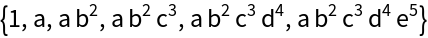#Function Repository Resource:

# FoldIndexedList

Perform a folding operation while also parsing the index as the third argument

Contributed by: Sander Huisman  |  SHuisman
 ResourceFunction["FoldIndexedList"][f,x,{a,b,…}] gives {x,f[x,a,1],f[f[x,a,1],b,2],…}. ResourceFunction["FoldIndexedList"][f,{a,b,c,…}] gives {a,f[a,b,1],f[f[a,b,1],c,2]}. ResourceFunction["FoldIndexedList"][f] represents an operator form of ResourceFunction["FoldIndexedList"] that can be applied to expressions.

## Details and Options

With a length n list, ResourceFunction["FoldIndexedList"] generates a list of length n+1.
The head of list in ResourceFunction["FoldIndexedList"][f,x,list] need not be List.
ResourceFunction["FoldIndexedList"][f][list] is equivalent to ResourceFunction["FoldIndexedList"][f,list].
ResourceFunction["FoldIndexedList"][f][x,list] is equivalent to ResourceFunction["FoldIndexedList"][f,x,list].

## Examples

### Basic Examples (1)

Map a function over a list including the index:

 In:=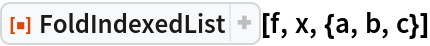Out=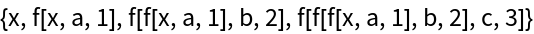### Scope (2)

Without an initializer, the first element of list is the initializer:

 In:=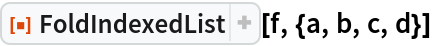Out=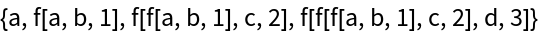Make an operator:

 In:=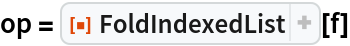Out=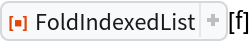Feed a single list to the operator:

 In:=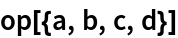Out=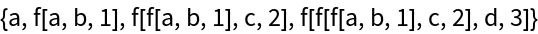Feed an initializer and a list to the operator:

 In:=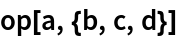Out=### Applications (3)

Form a continued fraction with increasing power:

 In:=Out=Form a continued fraction with an increasing numerator:

 In:=Out=Form the product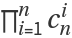:

 In:=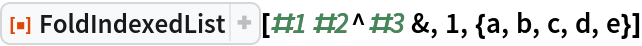Out=